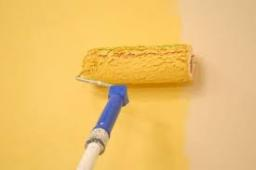# Painting school

Redecoration school had scheduled for four crew to 10 days. After two days, a single worker was ill. For how many days was the school painted when remain workers working with the same tempo?

x =  12.6667 d

### Step-by-step explanation:

(2·4)/(4·10)+((x-2)·3)/(4·10)=1

0.075x = 0.95

x = 0.95/0.075 = 12.66666667

x = 38/3 ≈ 12.666667

Our simple equation calculator calculates it.Did you find an error or inaccuracy? Feel free to write us. Thank you!

Tips for related online calculators
Do you have a linear equation or system of equations and looking for its solution? Or do you have a quadratic equation?
Do you want to convert time units like minutes to seconds?